## Difference Between Ambiguous and Unambiguous Grammar-

Before you go through this article, make sure that you have gone through the previous article on Ambiguous Grammar.

Some of the important differences between ambiguous grammar and unambiguous grammar are-

 Ambiguous Grammar Unambiguous Grammar A grammar is said to be ambiguous if for at least one string generated by it, it produces more than one-parse treeor derivation treeor syntax treeor leftmost derivationor rightmost derivation A grammar is said to be unambiguous if for all the strings generated by it, it produces exactly one-parse treeor derivation treeor syntax treeor leftmost derivationor rightmost derivation For ambiguous grammar, leftmost derivation and rightmost derivation represents different parse trees. For unambiguous grammar, leftmost derivation and rightmost derivation represents the same parse tree. Ambiguous grammar contains less number of non-terminals. Unambiguous grammar contains more number of non-terminals. For ambiguous grammar, length of parse tree is less. For unambiguous grammar, length of parse tree is large. Ambiguous grammar is faster than unambiguous grammar in the derivation of a tree.(Reason is above 2 points) Unambiguous grammar is slower than ambiguous grammar in the derivation of a tree. Example- E → E + E / E x E / id(Ambiguous Grammar) Example-E → E + T / TT → T x F / FF → id(Unambiguous Grammar)

Next Article- Algorithm To Check Grammar Ambiguity

Get more notes and other study material of Theory of Automata and Computation.

Watch video lectures by visiting our YouTube channel LearnVidFun.

## Grammar in Automata-

Before you go through this article, make sure that you have gone through the previous article on Types of Grammar in Automata.

On the basis of number of derivation trees, grammars are classified as-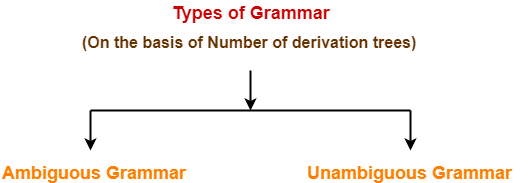1. Ambiguous Grammar
2. Unambiguous Grammar

## 1. Ambiguous Grammar-

A grammar is said to ambiguous if for any string generated by it, it produces more than one-

• Parse tree
• Or derivation tree
• Or syntax tree
• Or leftmost derivation
• Or rightmost derivation

### Example-

Consider the following grammar-

E → E + E / E x E / id

Ambiguous Grammar

This grammar is an example of ambiguous grammar.

Any of the following reasons can be stated to prove the grammar ambiguous-

### Reason-01:

Let us consider a string w generated by the grammar-

w = id + id x id

Now, let us draw the parse trees for this string w.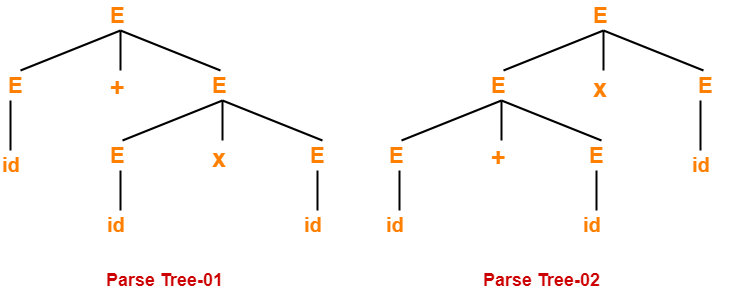Since two parse trees exist for string w, therefore the grammar is ambiguous.

### Reason-02:

Let us consider a string w generated by the grammar-

w = id + id x id

Now, let us draw the syntax trees for this string w.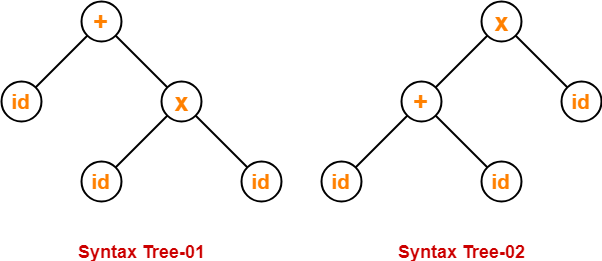Since two syntax trees exist for string w, therefore the grammar is ambiguous.

### Reason-03:

Let us consider a string w generated by the grammar-

w = id + id x id

Now, let us write the leftmost derivations for this string w.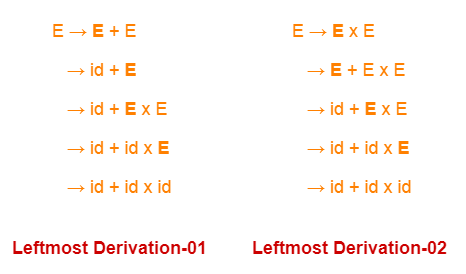Since two leftmost derivations exist for string w, therefore the grammar is ambiguous.

### Reason-04:

Let us consider a string w generated by the grammar-

w = id + id x id

Now, let us write the rightmost derivations for this string w.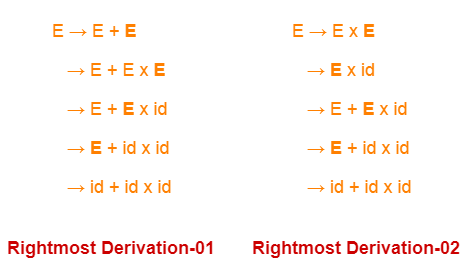Since two rightmost derivations exist for string w, therefore the grammar is ambiguous.

## 2. Unambiguous Grammar-

A grammar is said to unambiguous if for every string generated by it, it produces exactly one-

• Parse tree
• Or derivation tree
• Or syntax tree
• Or leftmost derivation
• Or rightmost derivation

### Example-

Consider the following grammar-

E → E + T / T

T → T x F / F

F → id

Unambiguous Grammar

This grammar is an example of unambiguous grammar.

To gain better understanding about Ambiguous Grammar,

Watch this Video Lecture

Next Article- Recursive Grammar

Get more notes and other study material of Theory of Automata and Computation.

Watch video lectures by visiting our YouTube channel LearnVidFun.

## Grammar in Automata-

### Formal Definition-

A Grammar is a 4-tuple such that-

G = (V , T , P , S)

where-

• V = Finite non-empty set of non-terminal symbols
• T = Finite set of terminal symbols
• P = Finite non-empty set of production rules
• S = Start symbol

## Grammar Constituents-

A Grammar is mainly composed of two basic elements-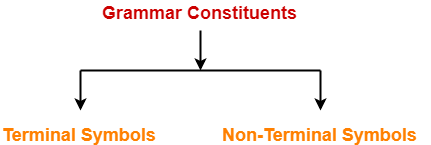1. Terminal symbols

2. Non-terminal symbols

### 1. Terminal Symbols-

• Terminal symbols are those which are the constituents of the sentence generated using a grammar.
• Terminal symbols are denoted by using small case letters such as a, b, c etc.

### 2. Non-Terminal Symbols-

• Non-Terminal symbols are those which take part in the generation of the sentence but are not part of it.
• Non-Terminal symbols are also called as auxiliary symbols or variables.
• Non-Terminal symbols are denoted by using capital letters such as A, B, C etc.

## Examples of Grammar-

### Example-01:

Consider a grammar G = (V , T , P , S) where-

• V = { S }                                                  // Set of Non-Terminal symbols
• T = { a , b }                                              // Set of Terminal symbols
• P = { S → aSbS , S → bSaS , S → ∈ }   // Set of production rules
• S = { S }                                                   // Start symbol

This grammar generates the strings having equal number of a’s and b’s

### Example-02:

Consider a grammar G = (V , T , P , S) where-

• V = { S , A , B }                                                  // Set of Non-Terminal symbols
• T = { a , b }                                                        // Set of Terminal symbols
• P = { S → ABa , A → BB , B → ab , AA → b }  // Set of production rules
• S = { S }                                                            // Start symbol

## Types of Grammars-

Grammars are classified on different basis as-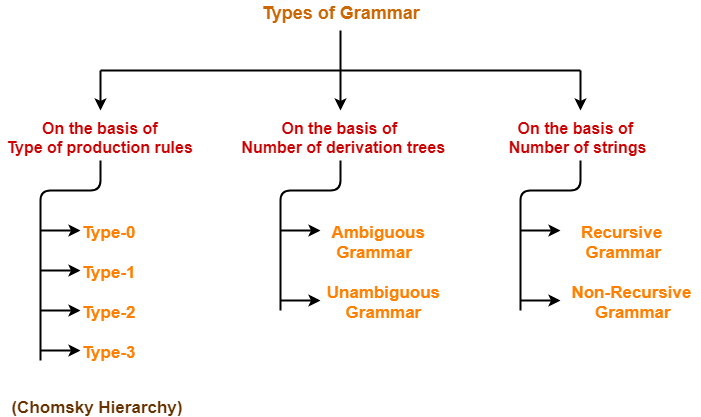We will discuss all these types of grammar one by one in detail.

## Equivalent Grammars-

 Two grammars are said to be equivalent if they generate the same languages.

### Example-

Consider the following two grammars-

S → aSb / ∈

#### Grammar G2-

S → aAb / ∈

A → aAb / ∈

Both these grammars generate the same language given as-

L = { anbn , n>=0 }

Thus, L(G1) = L(G2)

Since both the grammars generate the same language, therefore they are equivalent.

 ∴ G1 ≡ G2

To gain better understanding about Grammars in Automata,

Watch this Video Lecture

Next Article- Ambiguous Grammar

Get more notes and other study material of Theory of Automata and Computation.

Watch video lectures by visiting our YouTube channel LearnVidFun.